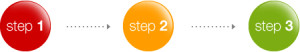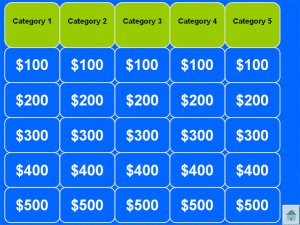# Three Types of Learning – Which One Do You Use? – Erik Kondo

There are three distinct categories of learning: Vertical Learning, Horizontal Learning, and Connective Learning.
Vertical Learning is the process of learning in a step by step linear fashion. You begin with the basics and build on the basics with successively more complicated concepts, skills, tasks, etc. Learning math is an example of this process. Additional and subtraction leads to algebra with leads to calculus, and so on. Vertical Learning is typical in the martial arts where basic skills are taught to beginning students and more advanced skills are taught to students that have mastered the basics. Colored belts that designate rank is typically a mark of Vertical Learning.

Step 1 -> Step 2 -> Step 3 -> Step 4Horizontal Learning is the process of learning additional concepts, skills, tasks, etc. that are independent of prior learning. Using math again, learning geometry is separate from learning statistics. One area does not necessarily build upon the other. In the martial arts, Horizontal Learning is seen by successive learning of different unrelated styles of martial arts. The person who has a belts in Karate, BJJ, Aikido, and Tai Chi is using Horizontal Learning.

Subject A + Subject B + Subject C + Subject DThe third method is Connective Learning. Connective Learning is the process of integrating and networking concepts, skills, tasks, etc. together to achieve understanding. Connective Learning is about building the understanding of the relationships between different concepts, skills, and tasks. The math example is seeing the relation of the common principles between geometry, statistics, and calculus. The martial artist that is able to relate the outwardly differing elements of various styles together to create unifying principles is using Connective Learning process.Intelligence is defined as the ability to acquire and apply knowledge and skills. Acquiring and applying is a form processing. Therefore, intelligence is the ability to process knowledge and skills. The brain is the body’s central processor. It uses an estimated 100 billion brain cells that each hold bits of information and communicate with each other to store information, knowledge, and execute tasks. The more efficiently these cells communicate (integrate/network) with each other the greater the processing power (intelligence).

Now imagine that Step 1, Step 2, Step 3, Step 4, Subject A, Subject B, Subject C, Subject D are all “bits of information” stored in Cells. Connective Learning is the process of interconnecting (integrating) these Cells together as efficiency as possible. If each connection is represented by a line, Vertical Learning uses three lines to connect four Steps. Horizontal Learning uses three lines to connect four Subjects. But Connective Learning is the process of developing the permutations (relationships, integrations, connectivity) between these Cells. How many different permutations can come from 8 different Cells? The answer is 40,320! Therefore, effective Connective Learning requires highlighting the “best” permutations.

Related Posts
Parts and Wholes – Rory Miller